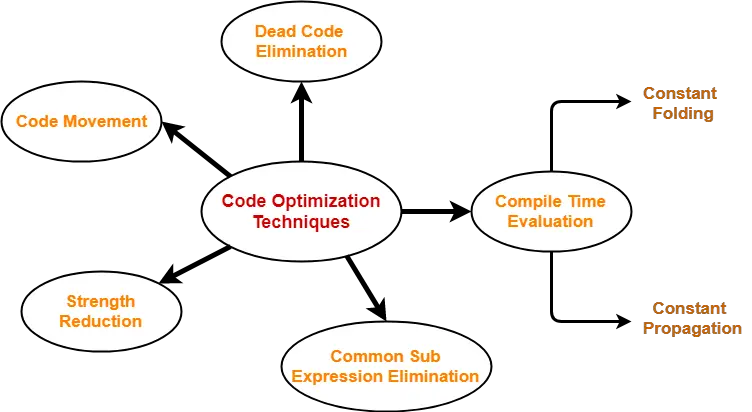# Code Optimization | Code Optimization Techniques

## Code Optimization-

 Code Optimization is an approach to enhance the performance of the code.

The process of code optimization involves-

• Eliminating the unwanted code lines
• Rearranging the statements of the code

The optimized code has the following advantages-

• Optimized code has faster execution speed.
• Optimized code utilizes the memory efficiently.
• Optimized code gives better performance.

## Code Optimization Techniques-

Important code optimization techniques are-1. Compile Time Evaluation
2. Common sub-expression elimination
4. Code Movement
5. Strength Reduction

## 1. Compile Time Evaluation-

Two techniques that falls under compile time evaluation are-

### A) Constant Folding-

In this technique,

• As the name suggests, it involves folding the constants.
• The expressions that contain the operands having constant values at compile time are evaluated.
• Those expressions are then replaced with their respective results.

### Example-

Circumference of Circle  = (22/7) x Diameter

Here,

• This technique evaluates the expression 22/7 at compile time.
• The expression is then replaced with its result 3.14.
• This saves the time at run time.

### B) Constant Propagation-

In this technique,

• If some variable has been assigned some constant value, then it replaces that variable with its constant value in the further program during compilation.
• The condition is that the value of variable must not get alter in between.

### Example-

pi = 3.14

Here,

• This technique substitutes the value of variables ‘pi’ and ‘radius’ at compile time.
• It then evaluates the expression 3.14 x 10 x 10.
• The expression is then replaced with its result 314.
• This saves the time at run time.

## 2. Common Sub-Expression Elimination-

 The expression that has been already computed before and appears again in the code for computation is called as Common Sub-Expression.

In this technique,

• As the name suggests, it involves eliminating the common sub expressions.
• The redundant expressions are eliminated to avoid their re-computation.
• The already computed result is used in the further program when required.

### Example-

 Code Before Optimization Code After Optimization S1 = 4 x i S2 = a[S1] S3 = 4 x j S4 = 4 x i  // Redundant  Expression S5 = n S6 = b[S4] + S5 S1 = 4 x i S2 = a[S1] S3 = 4 x j S5 = n S6 = b[S1] + S5

## 3. Code Movement-

In this technique,

• As the name suggests, it involves movement of the code.
• The code present inside the loop is moved out if it does not matter whether it is present inside or outside.
• Such a code unnecessarily gets execute again and again with each iteration of the loop.
• This leads to the wastage of time at run time.

### Example-

 Code Before Optimization Code After Optimization for ( int j = 0 ; j < n ; j ++) { x = y + z ; a[j] = 6 x j; } x = y + z ; for ( int j = 0 ; j < n ; j ++) { a[j] = 6 x j; }

In this technique,

• As the name suggests, it involves eliminating the dead code.
• The statements of the code which either never executes or are unreachable or their output is never used are eliminated.

### Example-

 Code Before Optimization Code After Optimization i = 0 ; if (i == 1) { a = x + 5 ; } i = 0 ;

## 5. Strength Reduction-

In this technique,

• As the name suggests, it involves reducing the strength of expressions.
• This technique replaces the expensive and costly operators with the simple and cheaper ones.

### Example-

 Code Before Optimization Code After Optimization B = A x 2 B = A + A

Here,

• The expression “A x 2” is replaced with the expression “A + A”.
• This is because the cost of multiplication operator is higher than that of addition operator.

To gain better understanding about Code Optimization Techniques,

Watch this Video Lecture

##Get more notes and other study material of Compiler Design.

Watch video lectures by visiting our YouTube channel LearnVidFun.

SummaryArticle Name
Code Optimization | Code Optimization Techniques
Description
In Compiler design, Code Optimization is an approach for enhancing the performance of the code. Various Code optimization techniques are- Compile Time Evaluation, Common Sub expression elimination, Code movement, Dead code elimination, Strength reduction.
Author
Publisher Name
Gate Vidyalay
Publisher Logo